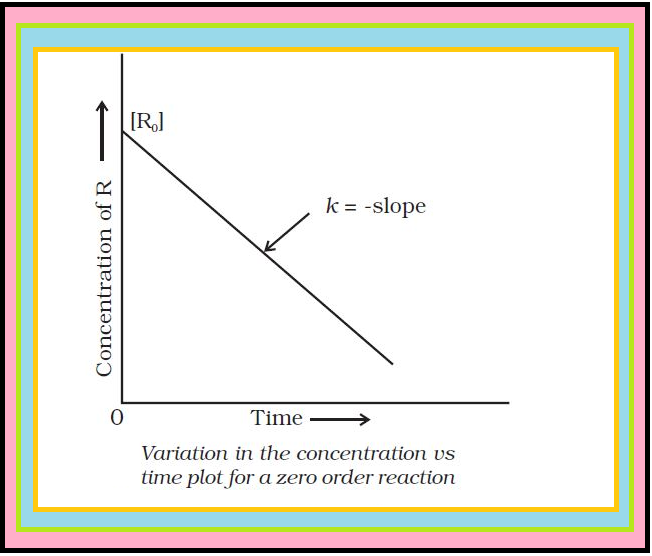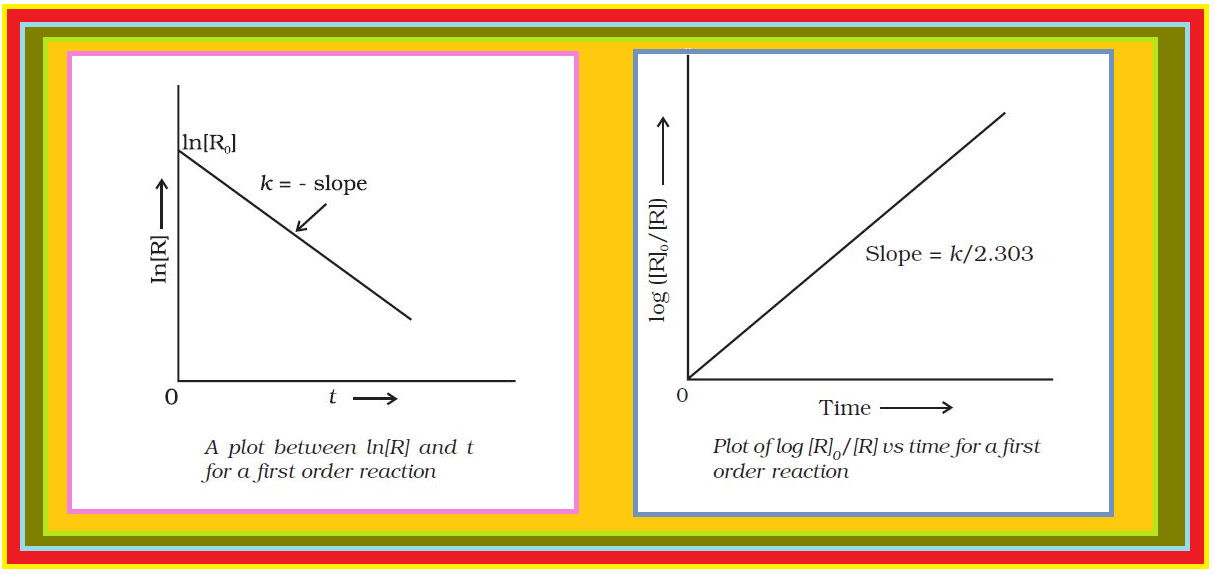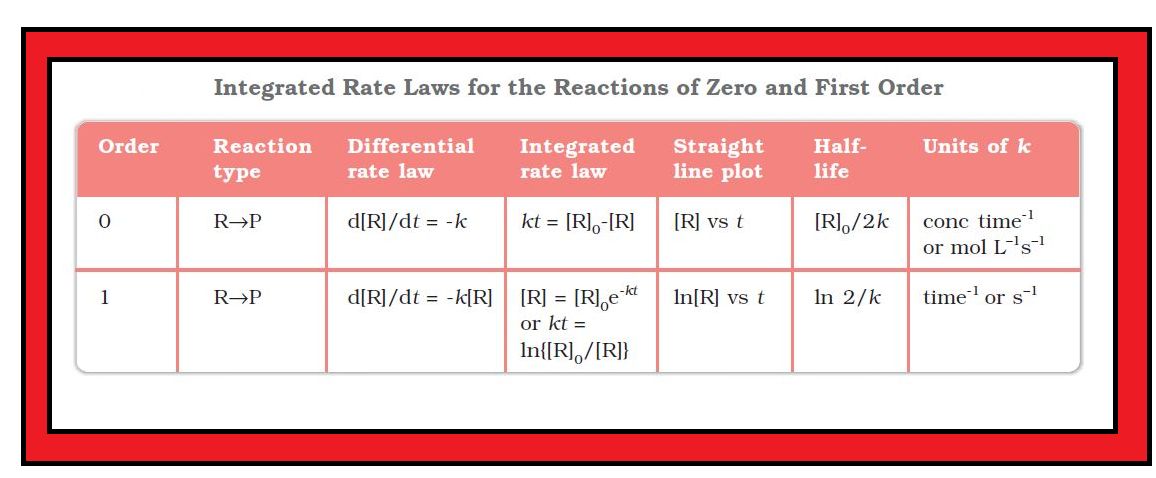Chemistry Integrated Rate Equations and Pseudo First Order Reaction
Click for Only Video

### Topics Covered :

● Integrated Rate Equations
● Zero Order Reaction
● First Order Reaction
● Half-life Period of a Reaction
● Pseudo First Order Reaction

### Integrated Rate Equations :

=> We know that the concentration dependence of rate is called differential rate equation.

=> It is not always convenient to determine the instantaneous rate, as it is measured by determination of slope of the tangent at point ‘t’ in concentration vs time plot. This makes it difficult to determine the rate law and hence the order of the reaction.

=> So, for avoiding this difficulty, we integrate the differential rate equation to give a relation between directly measured experimental data, i.e., concentrations at different times and rate constant.

=> The integrated rate equations are different for the reactions of different reaction orders. We shall determine these equations only for zero and first order chemical reactions.

### Zero Order Reactions :color{green}("Definition") : Zero order reaction means that the rate of the reaction is proportional to zero power of the concentration of reactants.

Consider the reaction :

color{red}(R → P)

color{red}(text(Rate) = (- d [ R])/(dt) = k [R]^0)

As any quantity raised to power zero is unity

color{red}(text(Rate) = - ( d [R])/(dt) = k xx 1)

color{red}(d [R] = - k dt)

Integrating both sides

color{red}([R] = - k t +I) ...............(1)

where, color{red}(I) is the constant of integration.

At color{red}(t = 0), the concentration of the reactant color{red}(R = [R]_0), where color{red}([R]_0) is initial concentration of the reactant. Substituting in equation (1)

color{red}([R]_0 = - k xx 0 + I)

color{red}([R]_0 = I)

Substituting the value of color{red}(I) in in the equation (1)

color{red}([R] = - k t + [R]_0) ............(2)

Comparing (2) with equation of a straight line, color{red}(y = mx + c),

if we plot color{red}([R]) against color{red}(t), we get a straight line (Fig.) with slope color{red}(= –k) and intercept equal to color{red}([R]_0).

Further simplifying equation (2), we get the rate constant, color{red}(k) as

color{red}(k = ( [R]_0 - [R])/t) .................(3)

=> Zero order reactions are relatively uncommon but they occur under special conditions.

=> Some enzyme catalysed reactions and reactions which occur on metal surfaces are a few examples of zero order reactions.

=> The decomposition of gaseous ammonia on a hot platinum surface is a zero order reaction at high pressure

color{red}(2NH_3 (g) underset (Pt catalyst ) overset(1130 K) → N_2 (g) +3H_2 (g)).

color{red}(text(Rate) = k [NH_3]^0 = k)

● In this reaction, platinum metal acts as a catalyst.

● At high pressure, the metal surface gets saturated with gas molecules. So, a further change in reaction conditions is unable to alter the amount of ammonia on the surface of the catalyst making rate of the reaction independent of its concentration.

=> The thermal decomposition of color{red}(HI) on gold surface is another example of zero order reaction.

### First Order Reactions :color{green}("Definition") : The rate of the reaction is proportional to the first power of the concentration of the reactant color{red}(R).

=> For example,

color{red}(R → P)

color{red}(text(Rate) = - (d [R])/(dt) = k [R] or color{red}((d [R])/([R]) = - k dt)

Integrating this equation, we get color{red}(ln [R] = - k t +I) ..........(4)

Again, color{red}(I) is the constant of integration and its value can be determined easily.

When color{red}(t = 0, R = [R]_0), where color{red}([R]_0) is the initial concentration of the reactant.

Therefore, equation (4) can be written as

color{red}(ln[R]_0 = - k xx 0+I)

color{red}(ln [R]_0 = I)

Substituting the value of color{red}(I) in equation (4)

color{red}(ln[R] = -k t + ln[R]_0) .........(5)

Rearranging this equation color{red}(ln \ \ ([R])/([R]_0) = - k t or color{red}(k = 1/t ln \ \ ([R]_0)/([R])) .........(6)

At time color{red}(t_1) from equation (5)

color{red}(text()^(**)ln [R]_1 = - k t_1 + text()^(**)ln [R]_0) .......(7)

At time color{red}(t_2),

color{red}(ln [R]_2 = -k t_2 + ln [R]_0) .............(8)

where, color{red}([R]_1) and color{red}([R]_2) are the concentrations of the reactants at time color{red}(t_1) and color{red}(t_2) respectively.

Subtracting (8) from (7)

color{red}(ln [R]_1 - ln [R]_2 = -k t_1 - ( -k t_2))

color{red}(ln \ \ ( [R]_1)/([R]_2) = k (t_2 - t_1))

color{red}(k = 1/(t_2-t_1) ln \ \ ([R]_1)/([R]_2)) ..........(9)

Equation (5) can also be written as :

color{red}(ln \ \ ([R])/([R]_0) = - k t)

Taking antilog of both sides color{red}([R] = [R]_0 e^(-k t)) ............(10)

Comparing equation (5) with color{red}(y = mx + c),

If we plot color{red}(ln [R]) against color{red}(t) we get a straight line with slope color{red}(= –k) and intercept equal to color{red}(ln [R]_0).

The first order rate equation can also be written in the form

color{red}(k = (2.303)/t log \ \ ([R]_0)/([R])) .............(11)

color{red}(text()^(**)log\ \ ([R]_0)/([R]) = (k t)/(2.303))

If we plot a graph between color{red}(log \ \ ([R]_0)/([R]) vs t), (Fig.), the slope color{red}(= k/2.303)

color{red}("Examples") : (i) Hydrogenation of ethene is an example of first order reaction.

color{red}(C_2H_4 (g) +H_2 (g) → C_2 H_6 (g))

color{red}(text(Rate) = k [C_2H_4])

(ii) All natural and artificial radioactive decay of unstable nuclei take place by first order kinetics.

color{red}(text()_(88)^(226)Ra → text()_(2)^(4)He +text()_(86)^(222)Rn)

color{red}(text(Rate) = k [Ra])

(iii) Decomposition of color{red}(N_2O_5) and color{red}(N_2O) are some more examples of first order reactions.

color{green}("First Order Gas Phase Reaction") :

Let us consider a typical first order gas phase reaction color{red}(A(g) → B (g) +C (g)).

=> Let color{red}(p_1) be the initial pressure of A and color{red}(p_t) the total pressure at time ‘color{red}(t’).

=> Integrated rate equation for such a reaction can be derived as Total pressure color{red}(p_t = p_A + p_B + p_C) (pressure units).

=> color{red}(p_A, p_B) and color{red}(p_C) are the partial pressures of A, B and C, respectively.

=> If x atm be the decrease in pressure of A at time t and one mole each of B and C is being formed, the increase in pressure of B and C will also be x atm each.

color{red}(tt(( , A(g) , -> ,B(g) , + , C(g) ), ( text{At} t =0 ,p_1atm , ,0 atm , , 0 atm ), ( text{At time } t , p_1 -x atm , , x atm , , x atm )))

where, color{red}(p_1) is the initial pressure at time color{red}(t = 0).

color{red}(p_t = (p_1-x) +x+x = p_1+x)

color{red}(x = (p_t - p_1))

where, color{red}(p_A = p_1-x = p_1 - (p_t - p_1))

 color{red}(= 2p_1-p_t)

color{red}(k = ((2.303)/t) (log \ \ p_1/p_A)) ...........(12)

color{red}( = (2.303)/t log \ \ p_1/(2p_1-p_t))

Q 2937680582The initial concentration of N_2O_5 in the following first order reaction N_2O_5(g) → 2 NO_2(g) + 1/2O_2 (g) was 1.24 × 10^(–2) mol L^(–1) at 318 K. The concentration of N_2O_5 after 60 minutes was 0.20 × 10^(–2) mol L^(–1). Calculate the rate constant of the reaction at 318 K.Solution:

For a first order reaction

log \ \ ([R]_1)/([R]_2) = (k (t_2-t_1))/(2.303)

k = (2.303)/((t_2-t_2)) log \ \ ([R]_1)/([R]_2)

 = (2.303)/(60 mi n - 0 mi n) log \ \ (1.24 xx 10^(-2) mol L^(-1))/(0.20 xx 10^(-2) mol L^(-1))

 = (2.303)/(60) log 62. mi n^(-1)

k = 0.0304 mi n^(-1)
Q 2967080885The following data were obtained during the first order thermal decomposition of N_2O_5 (g) at constant volume: 2N_2O_5 (g) → 2N_2 O_4 (g) +O_2 (g)

S. NO. Time/s Total Pressure / (atm)
1 0 0.5
2 100 0.512

Calculate the rate constant.Solution:

Let the pressure of N_2O_5 (g) decrease by 2x atm. As two moles of N_2O_5 decompose to give two moles of N_2O_4(g) and one mole of O_2(g) the pressure of N_2O_4(g) increases by 2x atm and that of O_2 (g) increases by x atm.

 2N_2O_5 (g) + O_2 (g) → 2N_2O_4 (g) Start t = 0 0.5 atm 0 atm 0 atm At time t (0.5 - 2x) atm 2x atm x atm

p_t = P_(N_2O_5) +P_(N_2O_4) +P_(O_2)

 = ( 0.5 - 2x) +2x+x = 0.5+x

x = p_t - 0.5

P_(N_2O_5) = 0.5 - 2x

 = 0.5 - 2(p_t - 0.5) = 1.5 - 2P_t

At t = 100s ; P_t = 0.512 atm

P_(N_2O_5) = 1.5-2xx0.512 = 0.476 atm

Using equation (4.16)

k = (2.303)/(100 s) xx 0.0216 = 4.98 xx 10^(-4) s^(-1)

### Half-Life of a Reaction :color{green}("Definition") : The half-life of a reaction is the time in which the concentration of a reactant is reduced to one half of its initial concentration. It is represented as color{red}(t_(1/2)).

color{green}("For the Zero Order Reaction") : Rate constant is given by equation3.

color{red}(k = ([R]_0 - [R])/t)

At color{red}(t = t_(1/2), [R] = 1/2 [R]_0)

The rate constant at color{red}(t_(1/2)) becomes color{red}(k = ([R]_0 - 1/2 [R]_0)/(t_(1/2)))

color{red}(t_(1/2) = ([R]_0)/(2k))

It is clear that color{red}(t_(1/2)) for a zero order reaction is directly proportional to the initial concentration of the reactants and inversely proportional to the rate constant.

color{green}("For the First Order Reaction") :

color{red}(k = (2.303 )/t log \ \ ([R]_0)/([R]))

at color{red}(t_(1/2) \ \ [R] = [R]_0/2)

So, the above equation becomes color{red}(k = (2.303)/t_(1/2) log \ \ ([R]_0)/([R]/2)) or color{red}(t_(1/2) = (2.303)/k log2))

color{red}(t_(1/2) = (2.303)/k xx 0.301)

color{red}(t_(1/2) = (0.693)/k) ..........(13)

=> For a first order reaction, half-life period is constant, i.e., it is independent of initial concentration of the reacting species.

=> The half-life of a first order equation is readily calculated from the rate constant and vice versa.

● For zero order reaction color{red}(t_(1/2) prop [R]_0), first order reaction color{red}(t_(1/2)) is independent of color{red}([R]_0).

Table summarises the mathematical features of integrated laws of zero and first order reactions.

Q 2937180982A first order reaction is found to have a rate constant, k = 5.5 × 10^(-14) s^(-1). Find the half-life of the reaction.Solution:

Half-life for a first order reaction is t_(1/2) = (0.693)/k

t_(1/2) = (0.693)/(5.5xx10^(-14) s^(-1)) = 1.26xx10^(14) s
Q 2937191082Show that in a first order reaction, time required for completion of 99.9% is 10 times of half-life (t_1/2) of the reaction.
When reaction is completed 99.9%, [R]_n = [R]_0 – 0.999[R]_0Solution:

k = (2.303)/t log \ \ ([R]_0)/([R])

 = (2.303)/t log \ \ ([R]_0)/([R]_0 - 0.999 [R]_0) = (2.303)/t \ \ log 10^3

t = 6.909 // k

For half-life of the reaction

t_(1/2) = 0.693 // k

t/t_(1/2) = (6.909)/k xx k/(0.693) = 10

### Pseudo First Order Reaction :

=> The order of a reaction is sometimes altered by conditions.

=> Consider a chemical reaction between two substances when one reactant is present in large excess.

color{red}("Examples") :

(i) During the hydrolysis of 0.01 mol of ethyl acetate with 10 mol of water, amounts of the various constituents at the beginning (t = 0) and completion (t) of the reaction are given as under :

color{red}(tt(( , CH_3COOC_2H_5 ,+, H_2O , overset(H^+)-> ,CH_3COOH , + , C_2H_5OH ), ( t =0 , 0.01 mol , ,10 mol , ,0 mol , , 0 mol ), ( t , 0 mol , , 9.9 mol , , 0.01 mol , , 0.01 mol )))

=> The concentration of water does not get altered much during the course of the reaction. So, in the rate equation

color{red}(text(Rate) = k' [CH_3 COOC_2H_5] [H_2O])

The term color{red}([H_2O]) can be taken as constant.

Thus, the equation becomes

color{red}(text(Rate) = k [CH_3COOC_2H_5])

where color{red}(k = k' [H_2O])

and the reaction behaves as first order reaction. Such reactions are called pseudo first order reactions.

(ii) Inversion of cane sugar is another pseudo first order reaction.

color{red}(undersettext(Cane sugar) (C_(12) H_(22) O_(11) ) + H_2O overset(H^+) →undersettext(Glucose) (C_6H_(12) O_6) + undersettext(Fructose) (C_6H_(12) O_6))

color{red}(text(Rate) = k [C_(12) H_(22) O_(11)])

Q 2927391281Hydrolysis of methyl acetate in aqueous solution has been studied by titrating the liberated acetic acid against sodium hydroxide. The concentration of the ester at different times is given below.

t/min 0 30 60 90
C//mol L^(-1) 0.8500 0.8004 0.7538 0.7096

Show that it follows a pseudo first order reaction, as the concentration of water remains nearly constant (55 mol L^(–1)), during the course of the reaction. What is the value of k′ in this equation? Rate = k′ [CH_3COOCH_3][H_2O]Solution:

For pseudo first order reaction, the reaction should be first order with respect to ester when [H_2O] is constant. The rate constant k for pseudo first order reaction is

k = (2.303)/t log \ \ C_0/C

where k = k' [H_2O]

From the above data we note

t/min C// mol L^(-1) k//mol L^(-1)
0 0.8500 -
30 0.8004 2.004 xx 10^(-3)
60 0.7538 2.002xx10^(-3)
90 0.7096 2.005xx10^(-3)

It can be seen that kappa [H_2O] is constant and equal to 2.004 × 10^(-3) mi n^(–1) and hence, it is pseudo first order reaction. We can now determine k from

k [H_2O] = 2.004xx10^(-3) mi n^(-1)

k [55 mol L^(-1) ] = 2.004 xx10^(-3) mi n^(-1)

k = 3.64xx10^(-5) mol^(-1) L mi n^(-1)